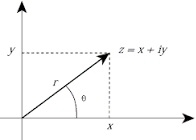# Python program for Complex Numbers

There are always two real roots of a positive number. For example, if x2 is 25, x is ±5. However, if x2 is -25 real roots do not exist. The square root of any negative number is the square root of its absolute value multiplied by an imaginary unit j = √−1.

Hence √−25 = √25 𝑋−1 = √25 × √−1 = 5j

A Complex number consists of real and imaginary component. It is represented as x+yj. Both x and y are real numbers. Y multiplied by imaginary unit forms an imaginary part of complex number.

Examples: 3+2j, 10-5.5J, 9.55+2.3j, 5.11e-6+4j

Python has a built-in complex data type. A complex number object can be created by literal representation as follows −

>>> x = 2+3j
>>> type(x)



The complex number object has two attributes real (returns the real component) and imag (returns imaginary component excluding imaginary unit j)

>>> x.real
2.0
>>> x.imag
3.0

It also has conjugate() method. Conjugate of a complex number has the same real component and imaginary component with the opposite sign. Hence conjugate of 2+3j is 2-3j

>>> x.conjugate()
(2-3j)

Python also has built-in complex() function which returns a complex number object. The function takes two parameters, one each for real and imaginary component. They can be of any numeric type (int, float or complex)

>>> complex(9,5)
(9+5j)
>>> complex(-6, -2.5)
(-6-2.5j)
>>> complex(1.5j, 2.5j)
(-2.5+1.5j)

If only one parameter is given it is treated as real component, imaginary component is assumed to be zero.

>>> complex(15)
(15+0j)

The function can also take a string as argument provided it contains numeric representation.

>>> complex('51')
(51+0j)
>>> complex('1.5')
(1.5+0j)

Addition and subtraction of complex numbers is similar to that of integers or floats. Real and imaginary parts are added / subtracted separately.

>>> a = 6+4j
>>> b = 3+6j
>>> a+b
(9+10j)
>>> a-b
(3-2j)

For multiplication consider complex number as a binomial and multiply each term in the first number by each term in the second number.

a = 6+4j
b = 3+2j
c = a*b
c = (6+4j)*(3+2j)
c = (18+12j+12j+8*-1)
c = 10+24j

In Python console the result verifies this −

>>> a = 6+4j
>>> b = 3+2j
>>> a*b
(10+24j)

Division of complex numbers is done as follows −

Let the two numbers be

a = 2+4j

b = 1-2j

and we want to calculate a/b.

Obtain conjugate of denominator which is 1+2j

Multiply numerator and denominator by the conjugate of the denominator to get result of division

c = a/b
c = (2+4j)*(1+2j)/(1-2j)(1+2j)
c = (2+4j+4j+8*-1)/(1+2j-2j-4*-1)
c = (-6+8j)/5
c = -1.2+1.6j

Following Python console session verifies above treatment.

>>> a = 2+4j
>>> b = 1-2j
>>> a/b
(-1.2+1.6j)

## The cmath module

Mathematical functions defined in math module of Python’s standard library process floating point numbers. For complex numbers, Python library contains cmath module.

Complex number z = x+yj is a Cartesian representation. It is internally represented in polar coordinates with its modulus r (as returned by built-in abs() function) and the phase angle Φ (pronounced as phi) which is counterclockwise angle in radians, between the x axis and line joining x with the origin. Following diagram illustrates polar representation of complex number −Functions in cmath module allow conversion of Cartesian representation to polar representation and vice versa.

polar() − This function returns polar representation of a Cartesian notation of complex number. The return value is a tuple consisting of modulus and phase.

>>> import cmath
>>> a = 2+4j
>>> cmath.polar(a)
(4.47213595499958, 1.1071487177940904)

Note that the modulus is returned by abs() function

>>> abs(a)
4.47213595499958

phase() − This function returns counterclockwise angle between x axis and segment joining a with origin. The angle is represented in radians and is between π and -π

>>> cmath.phase(a)
1.1071487177940904
z = x+yj
Φ

rect() − This function returns Cartesian representation of complex number represented in polar form i.e. in modulus and phase

>>> cmath.rect(4.47213595499958, 1.1071487177940904)
(2.0000000000000004+4j)

The cmath module contains alternatives for all mathematical functions defined in math module. There are trigonometric and logarithmic functions as explained below −

cmath.sin() − This function returns the sine trigonometric ratio for phase angle represented in radians.

>>> import cmath
>>> a = 2+4j
>>> p = cmath.phase(a)
>>> cmath.sin(p)
(0.8944271909999159+0j)

Similarly, functions for other ratios cos(), tan(), asin(), acos() and atan() are defined in cmath module.

cmath.exp() − Similar to math.exp(), this function returns ex where x is a complex number and e is 2.71828

>>> cmath.exp(a)
(-1.1312043837568135+2.4717266720048188j)

cmath.log10() − This function calculates log value of complex number taking base as 10

>>> a = 1+2j
>>> cmath.log10(a)
(0.3494850021680094+0.480828578784234j)

cmath.sqrt() − This function returns square root of complex number.

>>> cmath.sqrt(a)
(1.272019649514069+0.7861513777574233j)

In this article we learned important features of Python’s complex number data type and how arithmetic operations can be done on it. We also explored various functions defined in cmath module.